Open in App
Not now

# Class 9 NCERT Solutions- Chapter 11 Constructions – Exercise 11.1

• Last Updated : 15 Mar, 2022

### Question 1. Construct an angle of 90° at the initial point of a given ray and justify the construction.

Solution:

Steps of construction

1. Take a ray with initial point A.
2. Taking care center and same radius draw an Arc of a circle which intersect AB at C.
3. With C as Centre and the same radius, draw an arc intersecting the previous arc at E.
4. With E as Centre and the same radius draw an arc which intersects the arc drawn in step 2 at F.
5. With E as Centre and the same radius, draw another arc, intersecting the previous arc at G.
6. Draw the ray AG.
7. Then ∠BAG is the required angle 90°

Justification:

Join AE, CE, EF and AE, AF

AC = CE = AE      [ by construction]

∴ ACE is an equilateral Triangle

⇒ ∠CAE = 60°    —————–1

Similarly, AE = EF = AF

∴Triangle AEF is an equilateral Triangle

⇒ ∠EAF = 60°

Because AG bisects ⇒ ∠EAF

∴∠GAE = 1\2 = 30° = 30°————2

1+2

∴∠CAE + ∠GAE = 60°+30°

∠GAB=30°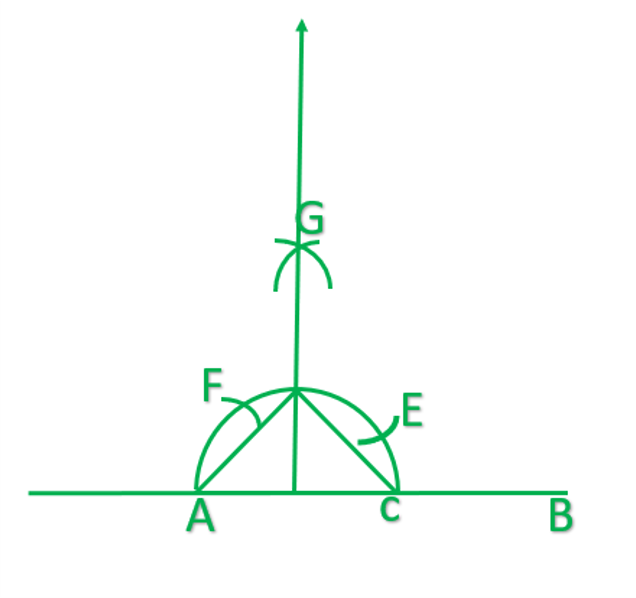### Question 2. Construct an angle of 45° at the initial point of a given ray and justify the construction.

Solution:

Step of Construction:

1. Take a ray AB with initial point A
2. Draw ∠BAF=90°
3. Taking C as Centre and radius more than   draw an arc.
4. Taking G as Centre and the same radius as before, draw another arc.
5. Taking G as Centre and the same radius as before, draw another arc. Intersecting previous arc at H.
6. Draw the ray AH.
7. Then ∠BAH is the required angle of 45°

Justification:

Join GH and HC    (construct)

In ∆ AHG and ∆ AHC

AH=AH………………[common]

AHG≅AHC             [S.S.S]

∠HAG=∠HAC                [C.P.C.T]

But ∠HAG+∠HAC=90

∠HAG=∠HAC=90\2=45

∴∠BAH=45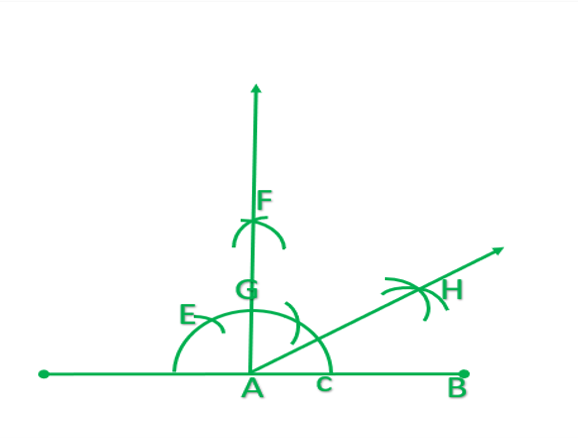### Question 3. Draw the angles of the following measurement

i) 30°

Solution:

Step of construction

1. Draw a ray AB with initial point A.
2. With A as centre, draw an arc intersecting AB at c.
3. With c as centre and the same radius, draw another arc, intersecting the previously drawn arc at D.
4. Taking C and D as centre  and with the radius more than 1\2 DC draws arcs to intersect each other at E.
5. Draw ray AE. ∠EAB is the required angle of 30.

ii) 22 ½°

Solution:

Steps of construction

1. Take a ray AB
2. Draw an angle ∠AB=90° on point A.
3. Bisect ∠CAB and draw ∠DAB=45°
4. Bisect ∠DAB and draw ∠EAB
5. ∠EAB is required angle of 22 ½°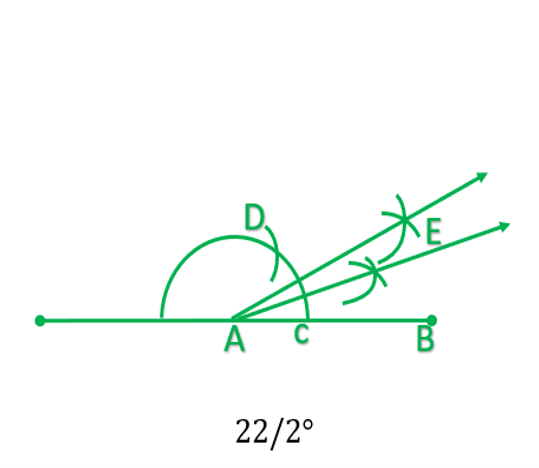iii) 15°

Solution:

Steps of construction

1. Take a ray AB.
2. Draw an arc on AB, by taking A a center, which intersect AB at c.
3. From C with the same radius draw another re which intersect the previous  arc at D.
4. Join DA.
5. ∠DAB =60°
6. Bisect  ∠DAB and draw angle  EAB=30°
7. Bisect ∠EAB and draw ∠FAB
8. ∠FAB is the required angle.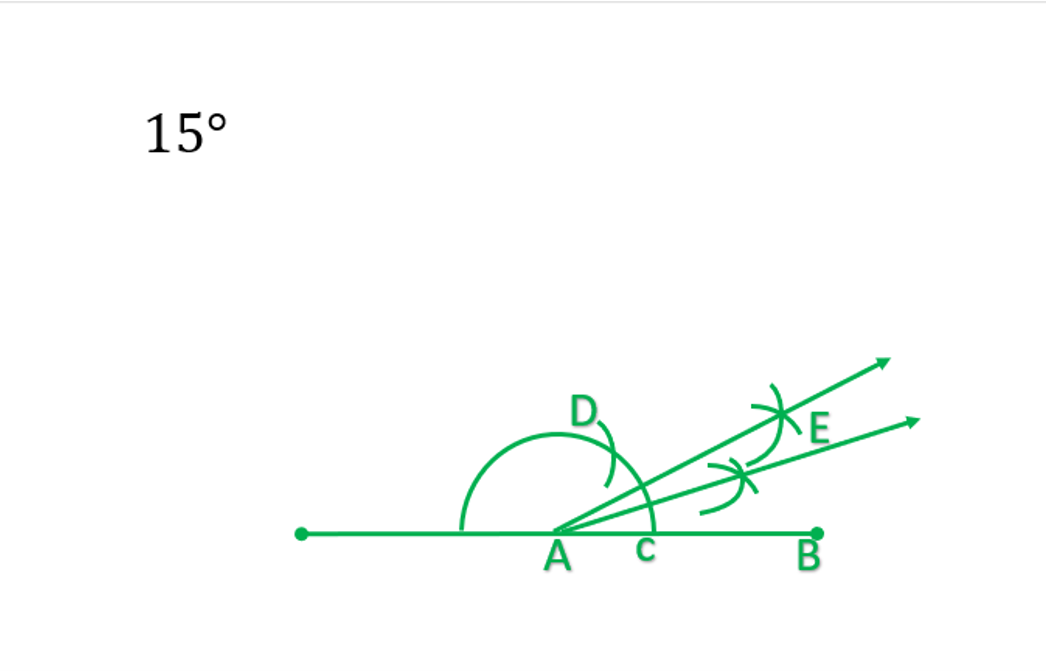### (i) 75°

Solution:

Steps of construction

1. Draw a ray AB with initial point  A.
2. At point A draw an angle ∠CAB=90°
3. At point A draw ∠DAB=60°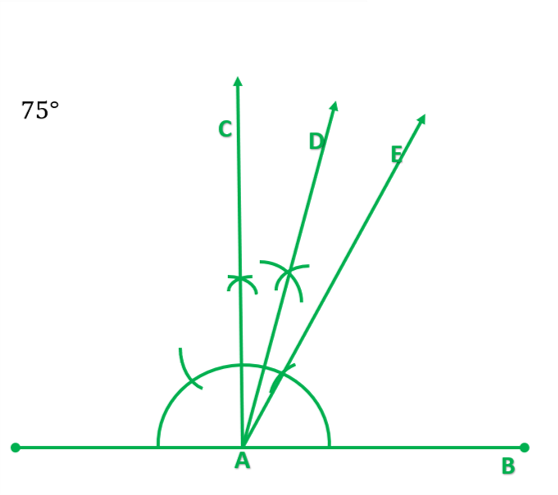### (ii) 105°

Solution:

Steps of construction

1. Draw a ray AB with initial point  A.
2. At point A draw an angle ∠CAB=90°
3. At point A draw ∠DAB=120°
5. ∠EAB=75°        {∠EAB=∠EAC+∠CAB=15°+90°=105°}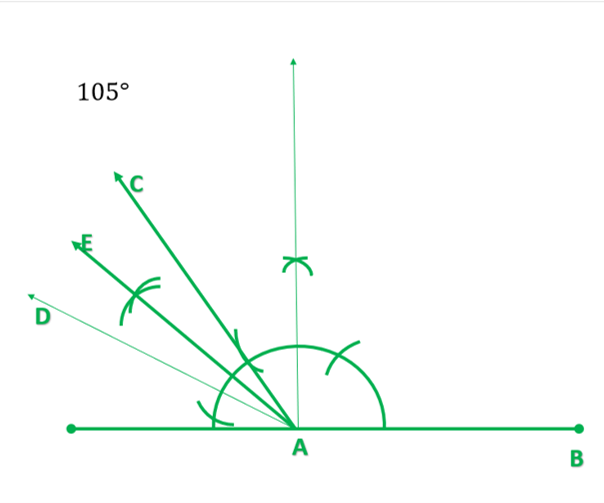### (iii) 135°

Solution:

Steps of construction

1. Draw a ray AB with initial point A.
2. At point A draw an angle ∠CAB=120°
3. At point A draw ∠DAB=150°
5. ∠EAB=135°        {∠EAB=∠EAC+∠CAB=15°+120°=135°}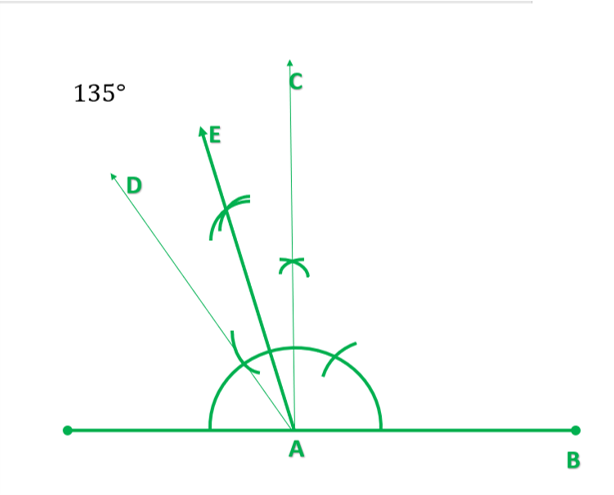### Question 5. Construct an equilateral triangle, given its side and justify the construction.

Solution:

Steps of construction

1. Draw a line segment of AB of a given length.
2. With A and B as centre and radius equal to AB draw arcs to intersect each other at c.
3. Join AC and BC.

Then ABC is the required equilateral triangle.

Justification:

AB=AC    ……………. [by construction]

AB=BC    ……………..[by construction]

AB=AC=BC

Hence, ∆ABC is required equilateral triangle.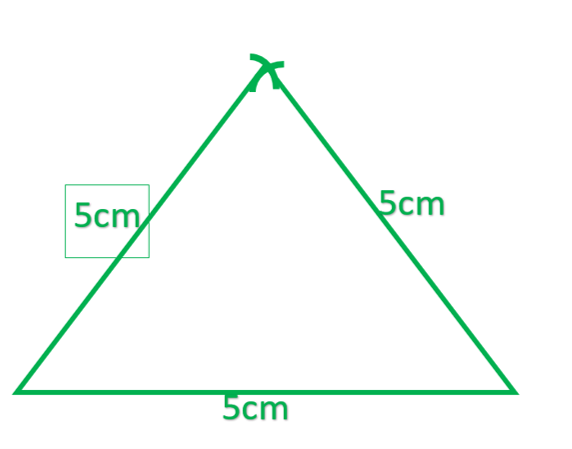My Personal Notes arrow_drop_up
Related Articles